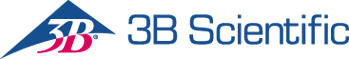3B Scientific

# 3b Scientific Experiment: Rotational Motion with Uniform Acceleration

## 3b Scientific Experiment: Rotational Motion with Uniform Acceleration

Regular price \$2,250.00
Regular price Sale price \$2,250.00
Sale Sold out

# Experiment: Rotational Motion with Uniform Acceleration

Objective: Confirm Newton’s equation of motion

For a body that rotates about a fixed axis with uniform acceleration, the angle of rotation φ increases in proportion to the square of the time t. From this proportionality factor it is possible to calculate the angular acceleration α, which in turn depends, according to Newton’s equation of motion, on the accelerating torque (turning moment) and the moment of inertia of the rigid body.